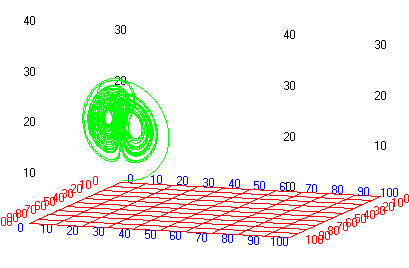# Lorenz strange attractors

In 1963 the meteorologist and mathematician E. N. Lorenz published solutions for a simplified mathematical model of atmospheric turbulence in an air cell under a thunderhead. The model is now generally known as an illustration for chaotic behaviour and strange attractors.The basic equations are:

 d(x1)/dt = a*(x2-x1) d(x2)/dt = (r-x3)*x1-x2 d(x3)/dt = x1*x2-b*x3

where:

• x1 is the amplitude of the convective air currents
• x2 is the temperature difference between the rising and falling currents
• x3 is the deviation of temperature from the mean
• a, r and b are dimensionless physical parameters